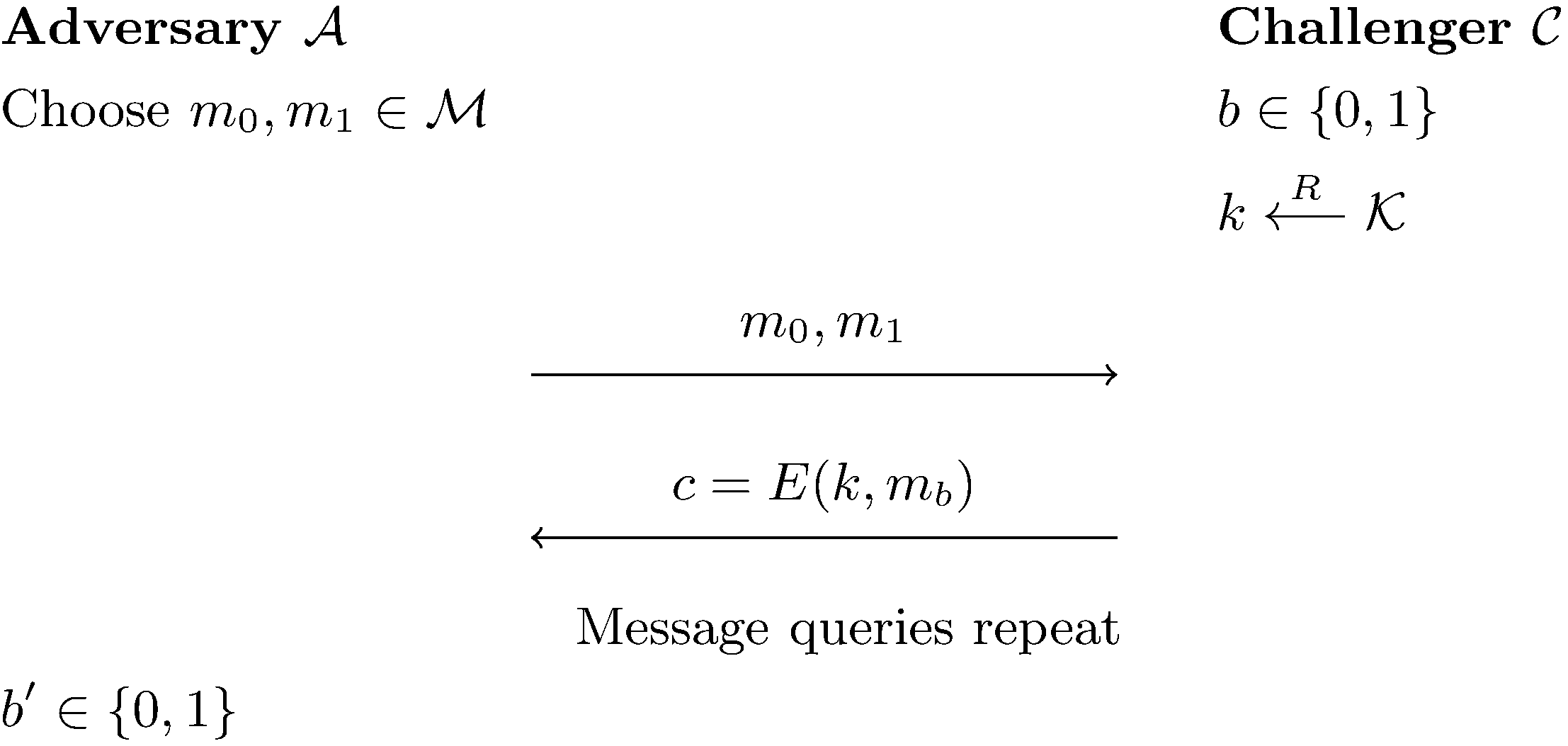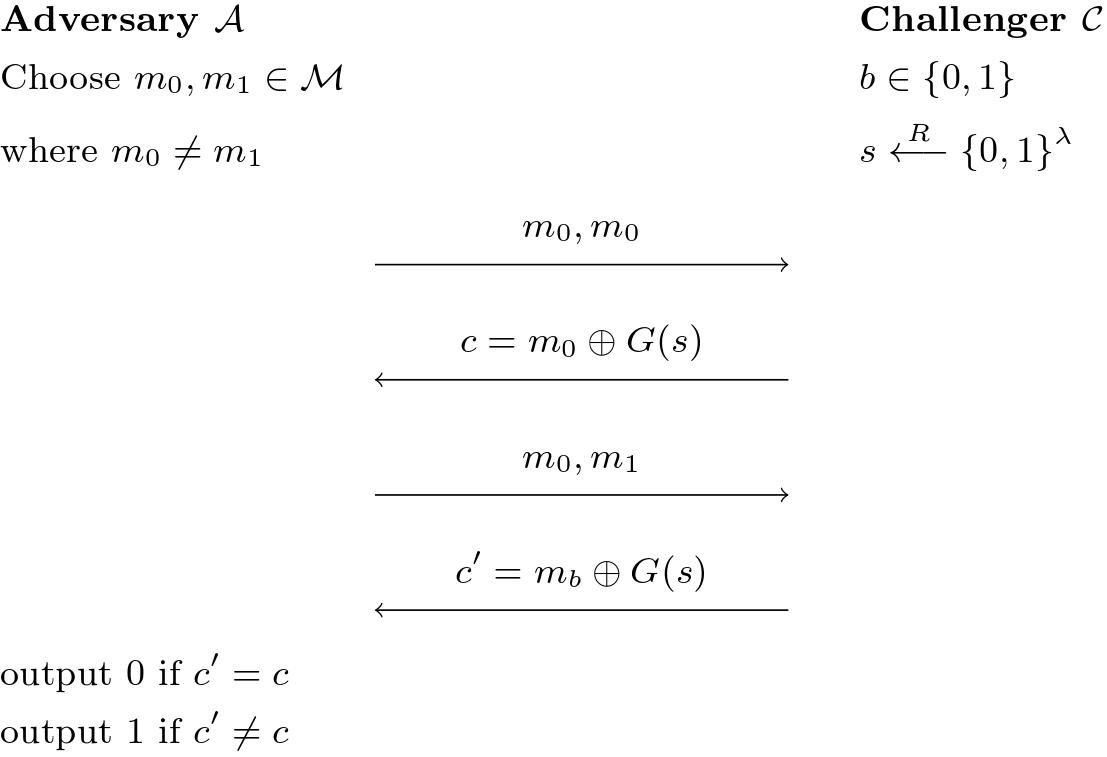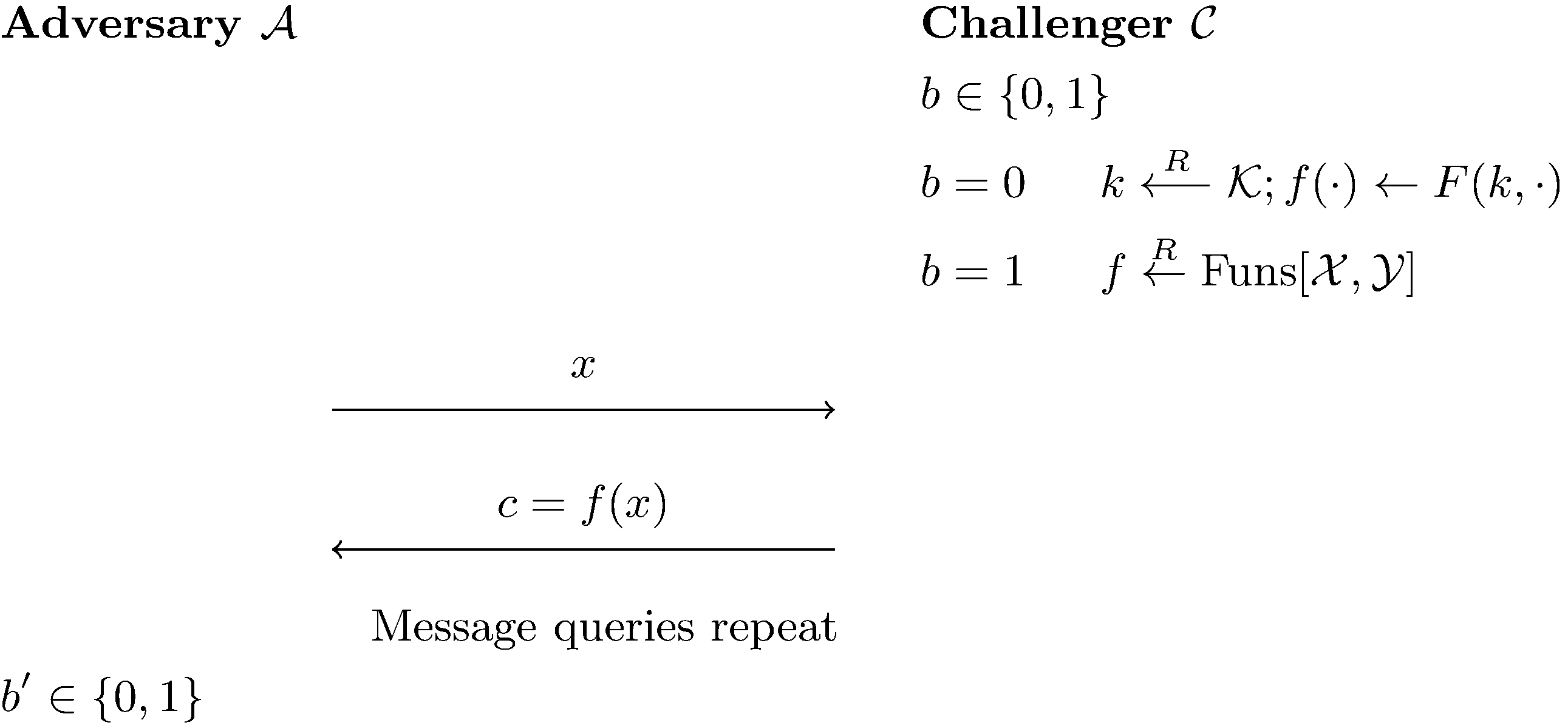Someone's Intermediate Representation

0%

All info comes from David Wu’s Lecture and Boneh-Shoup Book.

This note will be focusing on PRG security, PRF and Block Cipher.

Claim: If PRGs with non-trivial stretch ($n > \lambda$) exists, then $P\neq NP$.

Suppose $G :\lbrace 0,1\rbrace^\lambda \to \lbrace 0,1\rbrace^n$ is a secure PRG, consider the decision problem: on input $t\in \lbrace 0, 1\rbrace ^n$, does there exist $s \in \lbrace 0,1\rbrace^\lambda$ such that $t = G(s)$.

The reverse search of $G$ is in $NP$. If $G$ is secure, then no poly-time algorithm can solve this problem.

If there is a poly-time algorithm for the problem, it breaks PRG in advantage of $1 - \dfrac{1}{2^{n-\lambda}} > \dfrac{1}{2}$ since $n>\lambda$.

# CPA (Chosen Plaintext Attack) Security

Semantic Security still holds even if multiple ciphertext is seen by adversary.

The adversary can send multiple round of message query $m_0,m_1 \in \mathcal{M}$ to challenger and the challenger will send back one ciphertext $c_b$ with $k \overset{R}{\longleftarrow}\mathcal{K}$ and $b \in \lbrace 0, 1\rbrace$. The semantic security still holds.

CPA-Secure is defined by: An encryption scheme $\Pi_{SE} = (\text{Encryption},\text{Decryption})$ is CPA-Secure if $\forall \mathcal{A}$ efficient adversaries: $\text{CPAAdv}\lbrack \mathcal{A},\Pi_{SE}\rbrack = |\Pr\lbrack W_0 = 1\rbrack - \Pr\lbrack W_1 = 1\rbrack | = \text{negl}$.

$W_b$ means the experiment encrypt $m_b$ and $\mathcal{A}$’s guessing result.A stream cipher is not CPA-secure.• The query number is 2, a constant.
• $\Pr\lbrack \text{output}=1 \vert b=0\rbrack = 0$ and $\Pr\lbrack \text{output} = 1\vert b=1\rbrack = 1$, thus $\text{CPAAdv}\lbrack\mathcal{A},\Pi_{SE}\rbrack = 1 \gg\text{negl}$.

One thing we notice is that CPA-secure encryption is randomized rather than deterministic. Encrypting the same message twice should not reveal that identical messages were encrypted.

# PRF and PRP (Block Cipher)

Key crypto building block is called PRF (pseudo random function). A similar notion is PRP (pseudo random permutation), or block cipher.

Block cipher is an invertible keyed function: takes in a block of $n$ bits and outputs $n$ bits.

But it is not a encryption scheme, and it would end up with very broken stuff. It is a building block for secure encryption (maybe CPA secure or even more secure)

We define PRF by $F:\mathcal{K}\times\mathcal{X}\to\mathcal{Y}$ with key space $\mathcal{K}$ and domain $\mathcal{X}$ and range $\mathcal{Y}$ if $\forall \mathcal{A}$ efficient can have only $|W_0 - W_1| = \text{negl}$, where $W_b$ is the probability $\mathcal{A}$ outputs 1 in the experimentWe define $\text{PRFAdv}\lbrack\mathcal{A}, \text{F}\rbrack = |W_0 - W_1|$.

The size of $\text{Funs}\lbrack\mathcal{X,Y}\rbrack = |\mathcal{Y}|^{|\mathcal{X}|}$.

PRP is a $F:\mathcal{K}\times\mathcal{X}\to\mathcal{X}$ if

• $\forall k \in \mathcal{K}$, $F(k,\cdot)$ is a permutation on $\mathcal{X}$.
• $F^{-1}(k,\cdot)$ is efficient to compute $\forall x\in\mathcal{X},\forall k\in\mathcal{K}, F^{-1} (k,F(k,x))=x$.
• $k\overset{R}{\leftarrow} \mathcal{K}: F(k,\cdot)$ is computationally indistinguishable from $f(\cdot) \overset{R}{\leftarrow}\text{Perm}\lbrack \mathcal{X}\rbrack$. ($\text{Perm}\lbrack \mathcal{X}\rbrack$ is the set of all permutations on $\mathcal{X}$)

In this way, block cipher is another term of PRP.

# PRF implies PRG

Noticing that a block cipher has form $F:\lbrace 0,1\rbrace^\lambda \times \lbrace 0,1\rbrace ^n\to\lbrace 0,1\rbrace ^n$, we can define a PRG with $G:\lbrace 0,1\rbrace^\lambda \to \lbrace 0,1\rbrace^{ln}$, $G(k) = F(k,1)\parallel F(k,2) \parallel \cdots \parallel F(k,l)$.

Theorem: If $F$ is a secure PRF, then $G$ is a secure PRG.

We can construct the following experiment, say if $G$ is not secure, we can use it to break $F$.We can see that $\text{PRFAdv}\lbrack \mathcal{B},F\rbrack = |W_0 - W_1| = \text{PRGAdv}\lbrack \mathcal{A}, G\rbrack$.

$\mathcal{B}$ query is efficient by $\mathcal{A}$ efficient and $l$ polynomial. ($l < 2^n$ or $n > \log l$)

## PRF and PRP Distinctions

One thing we must see that the definition of PRF and PRP differ a little. For PRF, $f(1)=f(2)$ with probability $\dfrac{1}{2^n}$ but in PRP $f(1) = f(2)$ has no probability.

The adversary will not know until he sees a collision, or $f(x) = f(y)$.

PRF Switching Lemma: Let $F :\mathcal{K\times X \to X}$ be a secure PRP. For any $Q$ query adversary $\mathcal{A}$, $|\text{PRPAdv}\lbrack \mathcal{B},F\rbrack - \text{PRFAdv}\lbrack \mathcal{A}, G\rbrack| \leq \dfrac{Q^2}{2|\mathcal{X}|}$.

Since $\Pr\lbrack x ,y \overset{R}{\leftarrow}:x=y\rbrack = \dfrac{1}{|\mathcal{X}|}$. Also by $Q$ query takes $Q$ random points and compose $\dfrac{Q^2}{2}$ pairs, the total collision probability is $\dfrac{Q^2}{2|\mathcal{X}|}$.

For adversary, he should go $Q \sim \sqrt{|\mathcal{X}|}$, if $Q \ll \sqrt{|\mathcal{X}|}$ then it is safe to use PRF.

# PRF/PRP Reusable Encryption Scheme

In this way, PRP/PRF in “counter mode” gives us a stream cipher, but this is just one-time encryption scheme.

## Randomized Counter Mode

$$\newcommand{\da}{\bigg\downarrow\raise.5ex\rlap{\scriptstyle#1}} \begin{array}{ccccccccc} IV &&&& IV &&&& IV+1 &&&& \dots &&&& IV+l-1\newline &&&& \da{E_k} &&&& \da{E_k} &&&& &&&& \da{E_k}\newline &&m_1\longrightarrow&&\oplus&&m_2\longrightarrow&&\oplus&&&&&&m_{l-1}\longrightarrow&&\oplus\newline &&&&\da{}&&&&\da{}&&&&&&&&\da{}\newline IV &&&&c_1&&&&c_2&&&&\dots&&&&c_{l-1} \end{array}$$

We can choose a random starting point called initializing vector, and this is called randomized counter mode.

Theorem: Let $F : \mathcal{K\times X \to Y}$ be a secure PRF and $\Pi_{CTR}$ be randomized counter mode encryption scheme from $l$-block messages ($\mathcal{M=Y^{\leq l}}$).

Then $\forall\mathcal{A}$ efficient CPA adversaries, $\exists\mathcal{B}$ efficient PRF adversary
$$\text{CPAAdv}\lbrack\mathcal{A},\Pi_{CTR}\rbrack \leq \dfrac{4Q^2l}{|\mathcal{X}|} + 2\cdot \text{PRFAdv}\lbrack\mathcal{B},F\rbrack$$
The proof intuition can be considered:

• If there are no collision, or PRF never evaluate on a same block, then it is as if everything is encrypted under OTP.

• Collision event: $(x, x+1,\dots, x+l-1)$ overlaps with $(x’,x’+1,\dots,x’+l-1)$ when $x,x’\overset{R}{\longleftarrow}\mathcal{X}$, then the probability of collision is $\dfrac{2l}{|\mathcal{X}|}$.

The total possible pairs among $Q$ queries will be $\leq Q^2$, thus $\Pr\lbrack\text{collision}\rbrack \leq \dfrac{2lQ^2}{|\mathcal{X}|}$.

• Design 4 experiments with intermediate distributions:

• $W_0$: Encrypt $m_0$ with PRF
• $W_1$: Encrypt $m_0$ with OTP
• $W_2$: Encrypt $m_1$ with OTP
• $W_3$: Encrypt $m_1$ with PRF

Here we can see $|W_0 - W_1| = |W_2 - W_3| = \dfrac{2Q^2l}{|\mathcal{X}|} + \text{PRFAdv}\lbrack\mathcal{B},F\rbrack$, thus $|W_0 - W_3|$, which is CPA advantage, must satisfy $\text{CPAAdv}\lbrack\mathcal{A},\Pi_{CTR}\rbrack \leq \dfrac{4Q^2l}{|\mathcal{X}|} + 2\cdot \text{PRFAdv}\lbrack\mathcal{B},F\rbrack$.

### Nonce-Based Counter Mode

Divide $IV$ into two pieces: $IV = \text{nonce} \parallel \text{counter}$.

The only requirement here is that the nonce does not repeat in the encryption process.

Counter mode can be parallelized.

## Cipherblock Chaining (CBC Mode)

Cipherblock is chained up in the encryption session
$$\newcommand{\ra}{!!!!!!!!!!!!\xrightarrow{\quad#1\quad}!!!!!!!!} \newcommand{\da}{\bigg\downarrow\raise.5ex\rlap{\scriptstyle#1}} \begin{array}{ccccccccc} &&&&b_0&&&&b_1&&&&b_2&&&&\dots&&&&b_n\newline &&&&\Big\downarrow&&&&\Big\downarrow&&&&\Big\downarrow&&&&&&&&\Big\downarrow\newline IV&&\to&&\oplus&&&&\oplus&&&&\oplus&&&&&&&&\oplus\newline &&&&\da{E_k}&&\nearrow&&\da{E_k}&&\nearrow&&\da{E_k}&&\nearrow&&&&\nearrow&&\da{E_k}\newline &&&&c_0&&&&c_1&&&&c_2&&&&&&&&c_n \end{array}$$

and the structure of decryption of
$$\newcommand{\ra}{!!!!!!!!!!!!\xrightarrow{\quad#1\quad}!!!!!!!!} \newcommand{\da}{\bigg\downarrow\raise.5ex\rlap{\scriptstyle#1}} \begin{array}{ccccccccc} &&&&c_0&&&&c_1&&&&c_2&&&&\dots&&&&c_n\newline &&&&\da{E_k^{-1}}&&\searrow&&\da{E_k^{-1}}&&\searrow&&\da{E_k^{-1}}&&\searrow&&&&\searrow&&\da{E_k^{-1}}\newline IV&&\to&&\oplus&&&&\oplus&&&&\oplus&&&&&&&&\oplus\newline &&&&\da{}&&&&\da{}&&&&\da{}&&&&&&&&\da{}\newline &&&&b_0&&&&b_1&&&&b_2&&&&&&&&b_n \end{array}$$
Theorem: Let $\mathcal{K\times X\to Y}$ be a secure PRF and $\Pi_{CBC}$ be CBC encryption scheme for $l$-block message ($\mathcal{M = Y^{\leq l}}$).

Then $\forall\mathcal{A}$ efficient CPA adversaries, $\exists\mathcal{B}$ efficient PRF adversary
$$\text{CPAAdv}\lbrack\mathcal{A},\Pi_{CTR}\rbrack \leq \dfrac{2Q^2l^2}{|\mathcal{X}|} + 2\cdot \text{PRFAdv}\lbrack\mathcal{B},F\rbrack$$

The only difference here is that the collision between $Q$ intervals of $l$ length is changed to $Ql$ random blocks.